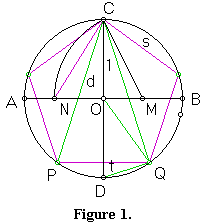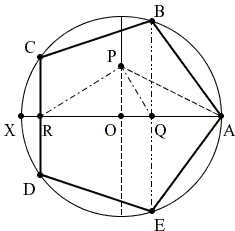# Regular Pentagon Inscribed in Circle by Paper Folding

Elsewhere at the site, S. Brodie described a construction of a regular pentagon inscribed in a given circle. Another - surprisingly simple - construction is due to H. W. Richmond. In this page, the two constructions are paraphrased in terms of paper folding a circle. For a mathematical justifications folding a circle needs a modified axiom O5, say O5':

For given points P1 and P2 and circle C, there exists a fold that passes through P1 and maps P2 into C.

One should also agree that points can be defined at the intersection of creases, i.e., straight lines; straight lines and circles and two circles.

### Construction 11. Fold a circle twice two create two perpendicular creases: diameters AB and CD. Point O is the center of the circle at the intersection of the two diameters.

2. Fold B onto O and mark point M at the intersection of the crease with OB.

3. Find a crease through M that places C on AB. C falls on N.

4. Find a crease through C that places N on the circle. N falls on E. CE is one side of a regular pentagon.

As an illustration for this construction, Linda Fahlberg-Stojanovska has prepared a screencast where she implements the construction with GeoGebra - free Java-based software.

### Construction 21. Form two perpendicular diameters AX and (say) UV. O is the center if the circle.

2. Fold U onto O to obtain P - the midpoint of OU.

3. Find the bisector of ∠APO by folding A onto OP while keeping P fixed. Let Q be the intersection of the bisector crease with AO.

4. Form a perpendicular through Q to AO. B and E are the intersections of the crease with the circle. B, A, E are successive vertices of a regular pentagon.

(Here is a screencast of following these instructions in GeoGebra.)Paper Folding Geometry